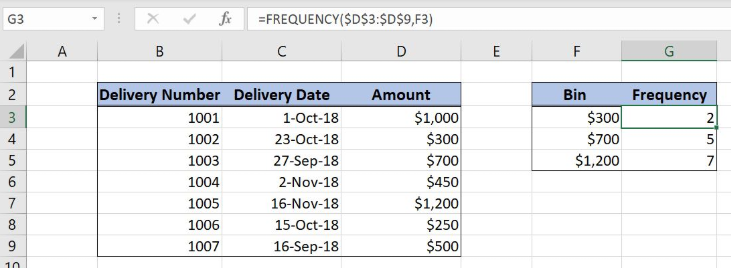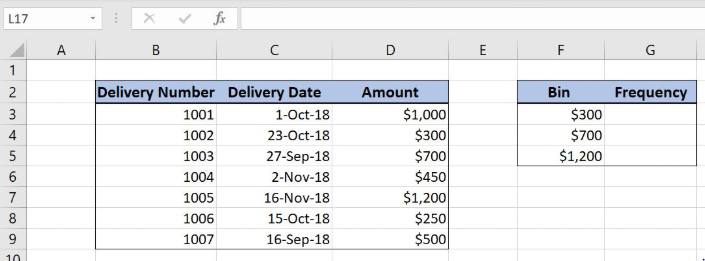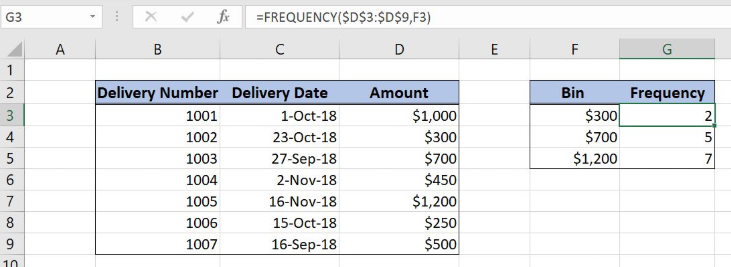Get instant live expert help with Excel or Google Sheets“My Excelchat expert helped me in less than 20 minutes, saving me what would have been 5 hours of work!”

#### Post your problem and you’ll get Expert help in seconds.

Your message must be at least 40 characters
Our professional Expert are available now. Your privacy is guaranteed.

# Excel FREQUENCY Function

Excel allows a user to check how many values from an array are less or equal than the limit, using the FREQUENCY function. This step by step tutorial will assist all levels of Excel users in counting values that meet a condition.Figure 1. The result of the FREQUENCY function

## Syntax of the FREQUENCY Formula

The generic formula for the FREQUENCY function is:

`=FREQUENCY(data_array, bins_array)`

The parameters of the FREQUENCY function are:

• data_array – an array with values
• bins_array – an array with bins limits

## Setting up Our Data for the FREQUENCY FunctionFigure 2. Data that we will use in the FREQUENCY example

Let’s look at the structure of the data we will use. Our data array consists of 3 columns: “Delivery Number” (column B), “Delivery Date” (column C) and “Amount” (column D). The bins array have 2 columns: “Bin” (column F) and “Frequency” (column G). In column G, we want to count how many values from column D are less or equal to the according Bin.

## Calculating the Frequency of a Value Using the FREQUENCY Function

In our example, we want to count how many values from column D are less or equal to the value from F3 (\$300). The result is in the cell G3.

The formula looks like:

`=FREQUENCY(\$D\$3:\$D\$9, F3)`

The data_array is the \$D\$3:\$D\$9 range, containing the values. The range must be fixed, as we are looking at the same range for every bin. The bins_array is F3 as we want to get the number of values from the column D less than F3.

To apply the FREQUENCY function, we need to follow these steps:

• Select cell G3 and click on it
• Insert the formula: `=FREQUENCY(\$D\$3:\$D\$9, F3:F9)`
• Press enter
• Drag the formula down to the other cells in the column by clicking and dragging the little “+” icon at the bottom-right of the cell.Figure 3. Using the FREQUENCY function to count values less than the limit

As we can see in Figure 3, rows 4 (\$300) and 8 (\$250) are less than \$300. Finally, the result in the cell G3 is 2.

Most of the time, the problem you will need to solve will be more complex than a simple application of a formula or function. If you want to save hours of research and frustration, try our live Excelchat service! Our Excel Experts are available 24/7 to answer any Excel question you may have. We guarantee a connection within 30 seconds and a customized solution within 20 minutes.

### Did this post not answer your question? Get a solution from connecting with the expert.Another blog reader asked this question today on Excelchat:## Subscribe to Excelchat.coAnother blog reader asked this question today on Excelchat: